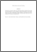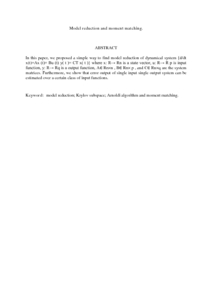# Model reduction and moment matching.

## Citation

Mohd, Ismail and Mamat, Mustafa and Dasril, Yosza and Ismail, Fudziah and Farikhin, , (2009) Model reduction and moment matching. Applied Mathematical Sciences, 3 (38). pp. 1849-1859. ISSN 1312-885X

## Abstract

In this paper, we proposed a simple way to find model reduction of dynamical system {d/dt x(t)=Ax (t)+ Bu (t) y( t )= CT x( t )} where x: R→ Rn is a state vector, u: R→ R p is input function, y: R→ Rq is a output function, A∈ Rn×n , B∈ Rn× p , and C∈ Rn×q are the system matrices. Furthermore, we show that error output of single input single output system can be estimated over a certain class of input functions.Preview
PDF (Abstract)
Model reduction and moment matching.pdfView Item